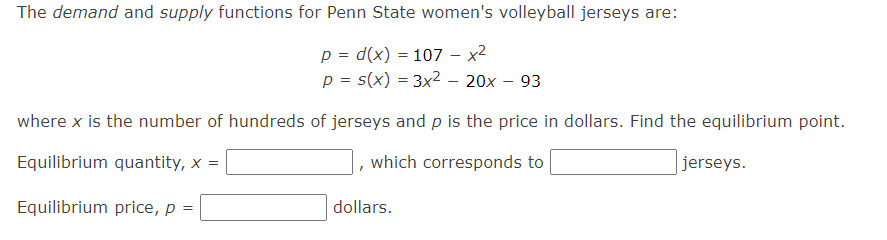Home / Expert Answers / Calculus / begin-array-l-p-d-x-107-x-2-p-s-x-3-x-2-20-x-93-end-array-where-x-is-the-pa902

# (Solved): $\begin{array}{l} p=d(x)=107-x^{2} \\ p=s(x)=3 x^{2}-20 x-93 \end{array}$ where $$x$$ is the ...$\begin{array}{l} p=d(x)=107-x^{2} \\ p=s(x)=3 x^{2}-20 x-93 \end{array}$ where $$x$$ is the number of hundreds of jerseys and $$p$$ is the price in dollars. Find the equilibrium point. Equilibrium quantity, $$x=$$ , which corresponds to jerseys. Equilibrium price, $$p=$$ dollars.

We have an Answer from Expert